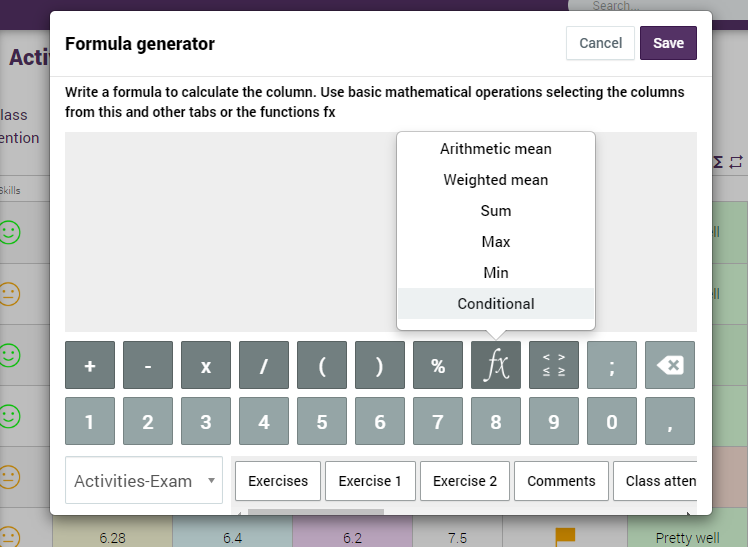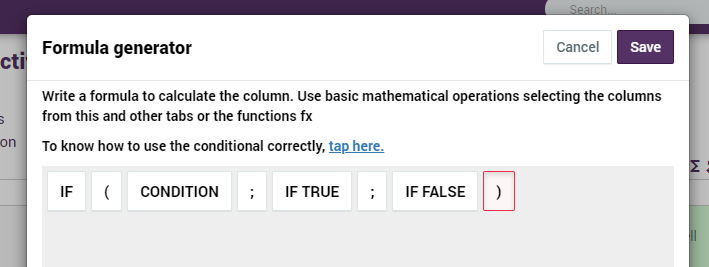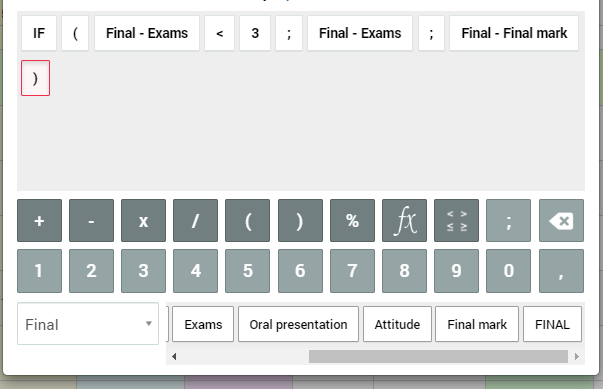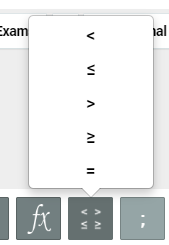# Applying conditionals

In some occasions, the value of an exam indicates us if the student can do average or not with other activities. Using Additio, we can do that with conditionals:

We can apply conditionals on calculated formulas once selected the calculus: Calculated formula. This will help us to put a rank of marks that will condition the final result.

To create them, create a column with a calculated formula and in fx, select the FX icon to configure the conditional.Then press the “conditional” option and it will appear a condition that you can adapt to make it accomplish the mark condition you want.Example:

In my lessons, if a student’s mark of an exam is lower than a 3, it does not make an average with the other activities, so it only counts the exam mark.

To configure the sections are the following ones: Condition (Here we will have to put the conditional that we want to apply), if it’s true(here we will have to write what happens if the exam mark is lower than 3) If it’s false (Here we will have to write what happens if the exam mark is higher than 3).

If EXAM <3; EXAM MARK; FINAL MARK

On this calculus, we can see that if the student’s mark is lower than 3 on an exam, the final mark will be just done with the exam mark, but if it is higher than 3, it makes an average with all the other activities.You will find the conditions to put higher or lower values with this option: